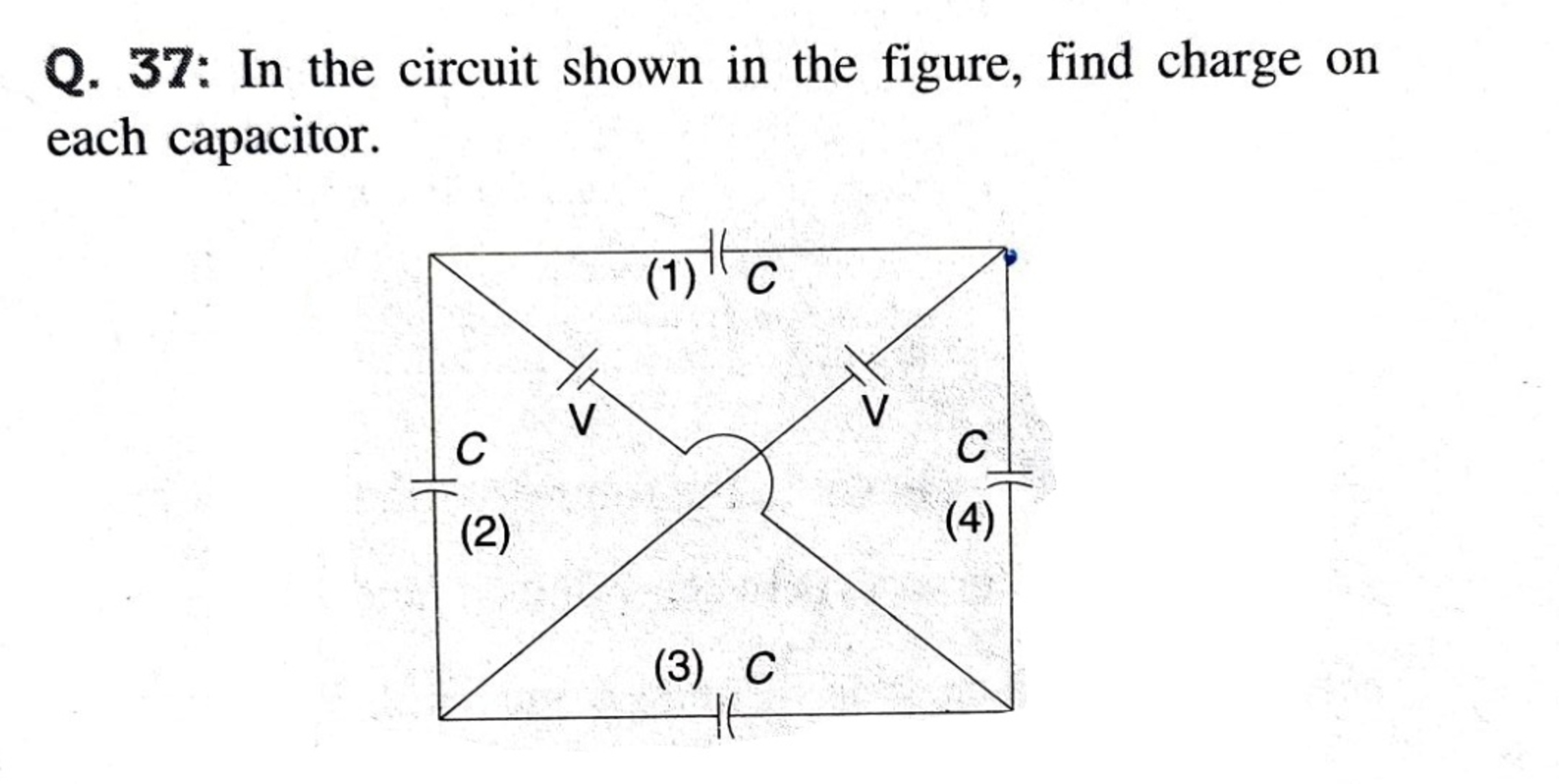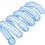# Voltages in Capacitor Network

Here is an interesting problem recently shared by Neeraj.As far as I can tell, there are infinitely many solutions, because when you write a system of four linear equations, the system matrix always ends up being singular. Let $V_1, V_2, V_3, V_4$ be the capacitor voltages. To get a solution, declare the value of $V_1$ and solve a system of three linear equations to get the other three voltages. The system of three linear equations is non-singular.

The following equations describe the system (being mindful of the capacitor polarities):

$V_2 - V_1 - V_4 + V_3 = 0 \\ V_2 + V_3 = V \\ V_4 - V_3 = V$

Since we are declaring the value of $V_1$, move it over to the other side:

$V_2 - V_4 + V_3 = V_1 \\ V_2 + V_3 = V \\ V_4 - V_3 = V$

Recall that $V$ is the battery voltage. For $V_1 = 0$, $(V_2, V_3, V_4) = (V,0,V)$. For $V_1 = 2V$, $(V_2, V_3, V_4) = (3 V,-2 V,-V)$. These are just two solutions out of infinitely many possibilities.

One other thing to note. The following relation also holds:

$V + V_1 - V + V_3 = 0 \\ V_1 + V_3 = 0$

So if $V_1$ is chosen as a positive value, $V_3$ will be negative. If one wishes to impose an additional constraint that none of the capacitor voltages can be negative, it follows that $V_1$ and $V_3$ are both zero.Note by Steven Chase
11 months, 4 weeks ago

This discussion board is a place to discuss our Daily Challenges and the math and science related to those challenges. Explanations are more than just a solution — they should explain the steps and thinking strategies that you used to obtain the solution. Comments should further the discussion of math and science.

When posting on Brilliant:

• Use the emojis to react to an explanation, whether you're congratulating a job well done , or just really confused .
• Ask specific questions about the challenge or the steps in somebody's explanation. Well-posed questions can add a lot to the discussion, but posting "I don't understand!" doesn't help anyone.
• Try to contribute something new to the discussion, whether it is an extension, generalization or other idea related to the challenge.

MarkdownAppears as
*italics* or _italics_ italics
**bold** or __bold__ bold
- bulleted- list
• bulleted
• list
1. numbered2. list
1. numbered
2. list
Note: you must add a full line of space before and after lists for them to show up correctly
paragraph 1paragraph 2

paragraph 1

paragraph 2

[example link](https://brilliant.org)example link
> This is a quote
This is a quote
    # I indented these lines
# 4 spaces, and now they show
# up as a code block.

print "hello world"
# I indented these lines
# 4 spaces, and now they show
# up as a code block.

print "hello world"
MathAppears as
Remember to wrap math in $$ ... $$ or $ ... $ to ensure proper formatting.
2 \times 3 $2 \times 3$
2^{34} $2^{34}$
a_{i-1} $a_{i-1}$
\frac{2}{3} $\frac{2}{3}$
\sqrt{2} $\sqrt{2}$
\sum_{i=1}^3 $\sum_{i=1}^3$
\sin \theta $\sin \theta$
\boxed{123} $\boxed{123}$

Sort by:

Notice how the $V_1 = 0$ case corresponds to the expected solution.

- 11 months, 4 weeks ago

The final comment that I just added seems relevant also. Perhaps the question assumes that all capacitor voltages must be positive, in which case their solution is indeed the only allowable one.

- 11 months, 4 weeks ago

@Steven Chase how do you know the polarities of capacitor?

- 11 months, 4 weeks ago

The curved side is negative, and the flat side is positive

- 11 months, 4 weeks ago

@Steven Chase now I am little bit more confused in this probelm.
Why we are doing $V_{1}=0$

- 11 months, 4 weeks ago

If all capacitor voltages must be positive (or at least not negative), $V_1$ has to be zero. See the last part of the note. Otherwise, it is just an arbitrary choice, given that the general four by four system is singular.

- 11 months, 4 weeks ago